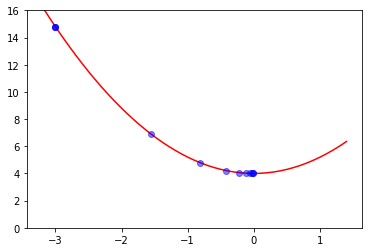Deep learning is so simple that it is hard to believe. Especially with how much magical it seems at first. At it's core is a piece of code that allows, one step at a time, to be just a bit closer to the solution. You can think of it like the way of finding the top of the mountain:Vocabulary:

• step - single step of the optimization algorithm that makes parameters of a function just a bit better
• gradient - the slope of the loss function, that we want to minimize
• learning rate - how fast we adjust parameters based on the gradient

### Task: Find minimum for $f: a x^2 + b$ with Gradient Descent

We have single variable here: $x$. With Gradient Descent we'll find the value where $f$ is minimal.

In short, gradient descent is an algorithm that walks down the slope - no more no less.

An example function $f$ in python looks like this:

def f(x):
return 1.2 * x**2 + 4


To compute gradient at where we are now, we will use PyTorch magic:

import torch



To walk along the function, we will change $x$ by the fraction of the gradient (gradient * learning_rate)

learning_rate = 0.2


and this is gradient descent algorightm in code:

def step(x, f):
# compute y at a given value of x
y = f(x)

# the backward function of PyTorch tensor computes gradients
y.backward()

# change value of x in a direction where function decreases
# (don't bother about x.data and x.grad = None - it is just necessary boilerplate)
x.data = x - learning_rate * x.grad.data

return x


gather x-es and y-s for 10 iterations

xypairs = []
for i in range(10):
xypairs.append([x.data, f(x)])
print(f"f({x:.2}) = {f(x):.2}")
x = step(x, f)

f(-3.0) = 1.5e+01
f(-1.6) = 6.9
f(-0.81) = 4.8
f(-0.42) = 4.2
f(-0.22) = 4.1
f(-0.11) = 4.0
f(-0.059) = 4.0
f(-0.031) = 4.0
f(-0.016) = 4.0
f(-0.0083) = 4.0

</input>And believe it or not, this is the building block of each the Neural Net - gradient descent algirithm. Stay tuned for the next post where we will use it on a MedicalNist dataset.